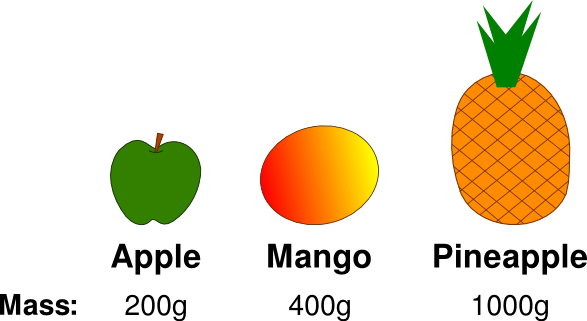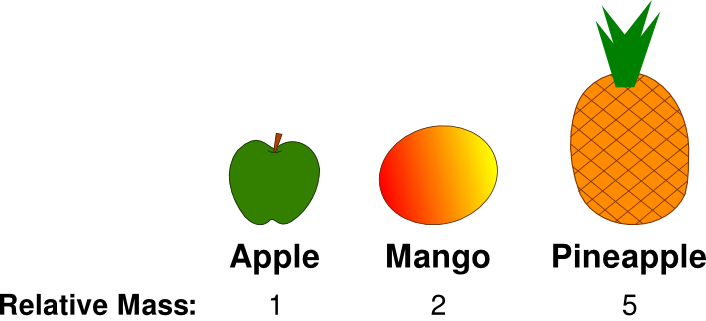Chemistry Foundations

1.3.3 - Relative Mass

# Relative Mass

Relative mass is a way of describing what the masses of different objects are compared to each other. Sometimes, we do not need to know the actual mass of an object, but just need to know how its mass compares to other objects. If that is the case then we can use relative mass.

## A relative mass example

Imagine we have an apple which has a mass of 200g, a mango which has a mass of 400g and a pineapple which has a mass of 1000g. This information is summarised below:We can see that the mango’s mass (400g) is two times the apple’s mass (200g).

We can also see that the pineapple’s mass (1000g) is five times the apple’s mass (200g).

Therefore, if we are only interested in how the masses compare to each other, we can simplify things by using relative mass. We can give the apple a relative mass of one, the mango a relative mass of two and the pineapple a relative mass of five. This is shown below:This shows that the mango’s mass is two times the apple’s mass and the pineapple’s mass is five times the apple’s mass.

## Relative mass is useful for comparisons

Using relative mass doesn’t tell us what the actual masses are - it only tells us how the masses compare to each other. There are many situations in Chemistry where we do not need to know the actual masses of particles, we only need to know how they relate to each other. Therefore, relative mass is very useful in Chemistry.

## Relative mass has no units

Note that relative mass does not have units. The pineapple’s relative mass is not five of anything, it is just five.

## Flashcards

Flashcards help you memorise information quickly. Copy each question onto its own flashcard and then write the answer on the other side. Testing yourself on these regularly will enable you to learn much more quickly than just reading and making notes.

1/2

What is relative mass?

2/2

What are the units of relative mass?